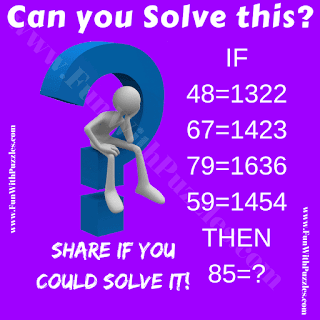## Thursday, July 26, 2018

Here is another tough logical Maths Question for adults. In this logical Maths Riddle, there are few logical statements given in terms of Mathematical equations. Assuming these equations are logical sequences, one has to find the logical pattern in these equations and then decode the value of the last equation. Can you solve this Logical Maths Question?Can you solve this Logical Maths Question?

1.